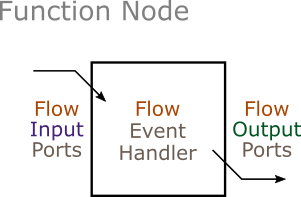# Function Nodes

The function node represents a single function that reads a set of input values and calculates a set of output values. It is placed in the dataflow network associated with the initialization or finalization phase of a composite node. In general, the purpose of a function node is to perform some or all of the data processing needed to either prepare parameter values for a simulation or extract statistics from the simulation.The function node base class is defined in function_node.h, which must be included by all function nodes.

``````#include <sydevs/systems/function_node.h>
``````

## Sample Declarations

An example of a function node is the `initial_position_node` class found in initial_position_node.h, which is part of the building7m demonstration project. Below is the class declaration, which inherits from `function_node`.

``````class initial_position_node : public function_node
{
public:
// Constructor/Destructor:
initial_position_node(const std::string& node_name, const node_context& external_context);
virtual ~initial_position_node() = default;

// Ports:
port<flow, input, std::pair<array2d<int64>, distance>> building_layout_input;
port<flow, output, array1d<int64>> initial_position_output;

private:
// Event Handlers:
virtual void flow_event();
};
``````

Another example is the `plus_node` (plus_node.h), which is part of the queueing project. This node is defined as a class template, allowing it to add together two values of any type. The user can obtain a concrete node type by specifying a type for `T` (e.g. `plus_node<int64>` or `plus_node<float64>`).

``````/**
* This node adds flow inputs "a" and "b" to produce flow output "c".
*/
template<typename T>
class plus_node : public function_node
{
public:
// Constructor/Destructor:
plus_node(const std::string& node_name, const node_context& external_context);
virtual ~plus_node() = default;

// Ports:
port<flow, input, T> a_input;
port<flow, input, T> b_input;
port<flow, output, T> c_output;

private:
// Event Handlers:
virtual void flow_event();
};
``````

## Constructor/Destructor Declarations

Constructors and destructor declarations are the same for all types of nodes (see Atomic Nodes – Constructor/Destructor Declarations).

## Ports

Function node ports are the same as ports on atomic nodes (see Atomic Nodes – Ports), except that function nodes use flow ports only.

## Constructor Definitions

Constructor definitions are the same as for atomic nodes (see Atomic Nodes – Constructor Definitions), except that the base class is `function_node`. Below is the example from initial_position_node.h

``````inline initial_position_node::initial_position_node(const std::string& node_name, const node_context& external_context)
: function_node(node_name, external_context)
, building_layout_input("building_layout_input", external_interface())
, initial_position_output("initial_position_output", external_interface())
{
}
``````

## Event Handlers

Function nodes must override the pure virtual member function `flow_event`.

``````    virtual void flow_event();
``````

### Flow Events

The `flow_event` function is invoked once, at any point after all flow input ports have values. These values are obtained using the port member function `value`. The event handler then processes the data and supplies values for all the flow output ports using the `assign` member function.

Below is the flow event handler from initial_position_node.h. It obtains as an input an array representing the layout of one floor of a simplified building. It then finds a single location inside the exterior walls of the building, and outputs the coordinates of that location.

``````inline void initial_position_node::flow_event()
{
array2d<int64> L = building_layout_input.value().first;
int64 nx = L.dims();
int64 ny = L.dims();
array1d<int64> pos({2}, {0, 0});
bool done = false;
for (int64 iy = 0; !done && iy < ny; ++iy) {
for (int64 ix = 0; !done && ix < nx; ++ix) {
if (L(ix, iy) == 0) {
// The first indoor grid cell has been found.
pos(0) = ix;
pos(1) = iy;
done = true;
}
}
}
if (!done) {
throw std::domain_error("The building has no indoor space.");
}
initial_position_output.assign(pos);
}
``````

The following flow event handler is from plus_node.h. It outputs the sum of the values of the inputs.

``````template<typename T>
inline void plus_node<T>::flow_event()
{
// Get the two flow input values, add them together, and assign the result
// to the flow output port.
const T& a = a_input.value();
const T& b = b_input.value();
T c = a + b;
c_output.assign(c);
}
``````